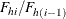The SURVEYSELECT Procedure

PPS Sequential Sampling

If you specify the METHOD=PPS_SEQ option, PROC SURVEYSELECT uses Chromy’s method of sequential random sampling. For more information, see Chromy (1979) and Williams and Chromy (1980). Chromy’s method selects units sequentially with probability proportional to size and with minimum replacement. Selection with minimum replacement means that the actual number of hits for a unit can equal the integer part of the expected number of hits for that unit, or the next largest integer. This can be compared to selection without replacement, where each unit can be selected only once, so the number of hits can equal 0 or 1. The other alternative is selection with replacement, where there is no restriction on the number of hits for each unit, so the number of hits can equal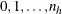, where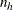is the stratum sample size.

Sequential random sampling controls the distribution of the sample by spreading it throughout the sampling frame or stratum, thus providing implicit stratification according to the order of units in the frame or stratum. You can use the CONTROL statement to sort the input data set by the CONTROL variables before sample selection. If you also use a STRATA statement, PROC SURVEYSELECT sorts by the CONTROL variables within strata. By default (or if you specify the SORT=SERP option), the procedure uses hierarchic serpentine ordering to sort the sampling frame by the CONTROL variables within strata. If you specify the SORT=NEST option, the procedure uses nested sorting. See the section Sorting by CONTROL Variables for descriptions of serpentine and nested sorting. If you do not specify a CONTROL statement, PROC SURVEYSELECT applies sequential selection to the observations in the order in which they appear in the input data set.

According to Chromy’s method of sequential selection, PROC SURVEYSELECT first chooses a starting unit randomly from the entire stratum, with probability proportional to size. The procedure uses this unit as the first one and treats the stratum observations as a closed loop. This is done so that all pairwise (joint) expected number of hits are positive and an unbiased variance estimator can be obtained. The procedure numbers observations sequentially from the random start to the end of the stratum and then continues from the beginning of the stratum until all units are numbered.

Beginning with the randomly chosen starting unit, Chromy’s method partitions the ordered stratum sampling frame intozones of equal size. There is one selection from each zone and a total ofhits (selections), although fewer thandistinct units might be selected. Beginning with the random start, the procedure accumulates the expected number of hits and computes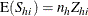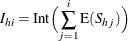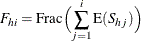where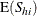represents the expected number of hits for unit i in stratum h,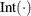denotes the integer part of the number, and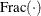denotes the fractional part.

Considering each unit sequentially, Chromy’s method determines the actual number of hits for unit i by comparing the total number of hits for the first (i – 1) units,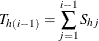with the value of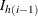.

If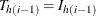, Chromy’s method determines the total number of hits for the first i units as follows. If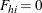or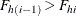, then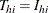. Otherwise,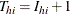with probability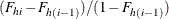And the number of hits for unit i is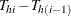.

If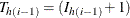, Chromy’s method determines the total number of hits for the first i units as follows. If, then. If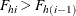, then. Otherwise,with probability